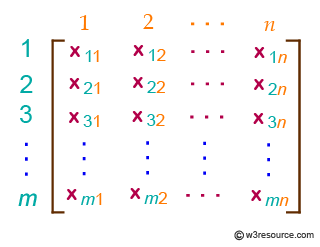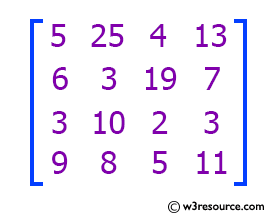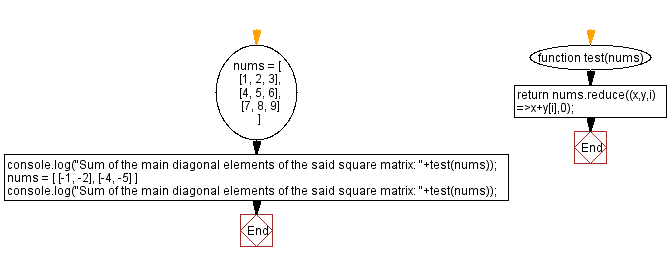# JavaScript: Sum of the main diagonal elements of a square matrix

## JavaScript Math: Exercise-85 with Solution

Write a JavaScript program to compute the sum of the main diagonal elements of a square matrix.

In mathematics, a matrix (plural matrices) is a rectangular array or table of numbers, symbols, or expressions, arranged in rows and columns, which is used to represent a mathematical object or a property of such an object.
An m × n matrix: the m rows are horizontal and the n columns are verticalIn mathematics, a square matrix is a matrix with the same number of rows and columns. An n-by-n matrix is known as a square matrix of order n. Any two square matrices of the same order can be added and multiplied.
Example:Test Data:
([ [1, 2, 3], [4, 5, 6], [7, 8, 9] ]) -> 15
( [ [-1, -2], [-4, -5] ]) -> -6

Sample Solution:

HTML Code:

``````<!DOCTYPE html>
<html>
<meta charset="utf-8">
<title>JavaScript program to Sum of the main diagonal elements of a square matrix</title>
<body>

</body>
</html>
```
```

JavaScript Code:

``````function test(nums) {
return nums.reduce((x,y,i)=>x+y[i],0);
}
nums = [
[1, 2, 3],
[4, 5, 6],
[7, 8, 9]
]
console.log("Sum of the main diagonal elements of the said square matrix: "+test(nums));
nums = [
[-1, -2],
[-4, -5]
]
console.log("Sum of the main diagonal elements of the said square matrix: "+test(nums));
```
```

Sample Output:

```Sum of the main diagonal elements of the said square matrix: 15
Sum of the main diagonal elements of the said square matrix: -6
```

Flowchart:Live Demo:

See the Pen javascript-math-exercise-85 by w3resource (@w3resource) on CodePen.

Improve this sample solution and post your code through Disqus

What is the difficulty level of this exercise?

Test your Programming skills with w3resource's quiz.

﻿